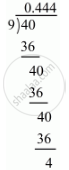# Express the Following Rational Number as Decimal: -4/9 - Mathematics

Express the following rational number as decimal:

-4/9

#### Solution

Given rational number is -4/9

Now we have to express this rational number into decimal form. So we will use long division methodTherefore, 4/9=0.444

rArr-4/9=-0.bar4

Hence, -4/9=-0.bar4

Concept: Introduction of Real Number
Is there an error in this question or solution?

#### APPEARS IN

RD Sharma Mathematics for Class 9
Chapter 1 Number Systems
Exercise 1.2 | Q 2.2 | Page 13
Share